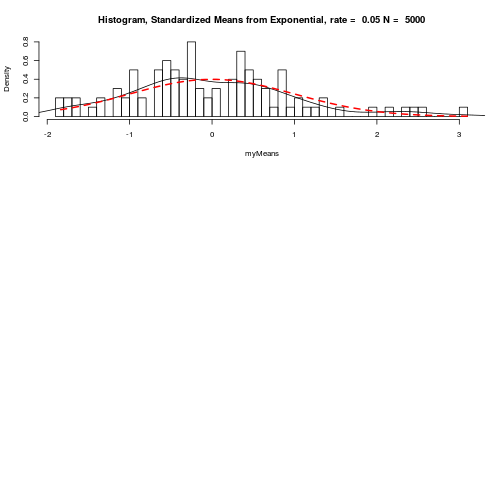``````###Paul Johnson
### April 14, 2008/ 2014-08-06

### Today, we were wondering about an exercise in Verzani that asks us to
### consider the sampling distribution of t

###  t = x-bar / std.dev(x)/sqrt(n)

### for a non-normal variable.  If x is Normal, this is exactly a t
### distribution, but if x is not Normal, then what?

### So if you drew a sample means from exponential or uniform
### distributions, and calculated

### x-bar / std.dev(x)/ sqrt(n)

### against which t distribution would one compare in order to
### decide if the distribution is similar to a t.

### My intuition was that the answer should be "the sample size"
### of x.

### In order to decide, I had to first get an idea of how the
### density of t changes with the df parameter.
### the following commands create a display of several t densities
### and a Normal overlaid on top.

## 2014-08-06
## I now prefer to save plots in pdf, and convert that to other
## formats like png or jpeg when necessary using ImageMagic. Some
## people don't have ImageMagick (poor souls) and they need to
## write direct from R to png or such. There are examples below.

SAVEME <- FALSE
pdf.options(height = 7, width = 7, onefile = TRUE, paper = "special", family = "Times")

x <- seq(0,4, length.out = 1000)
y <- matrix(0, ncol = 10, nrow = 1000)

y[,1] <- dt(x, df = 1)
y[,2] <- dt(x, df = 2)
y[,3] <- dt(x, df = 3)
y[,4] <- dt(x, df = 4)
y[,5] <- dt(x, df = 5)
y[,6] <- dt(x, df = 6)
y[,7] <- dt(x, df = 7)
y[,8] <- dt(x, df = 20)
y[,9] <- dt(x, df = 100)
y[,10] <- dt(x, df = 1000)

if (SAVEME) pdf(file = "normalAndTcompared-1.pdf")
matplot(x,y,  type = "l",ylab = "probability density")
lines(x, dnorm(x), col = 2, lty = 2, lwd = 3)
text(0, 0.25, "t,df = 1",pos = 4)
text(1, 0.33, "t,df = 1000")
text(.85, 0.28, "Normal(0,1)", pos = 4)
text(-0.2, 0.33, "t,df = 2", pos = 4)
title("Normal(0,1) and t(df = 1000) Coincide")
``````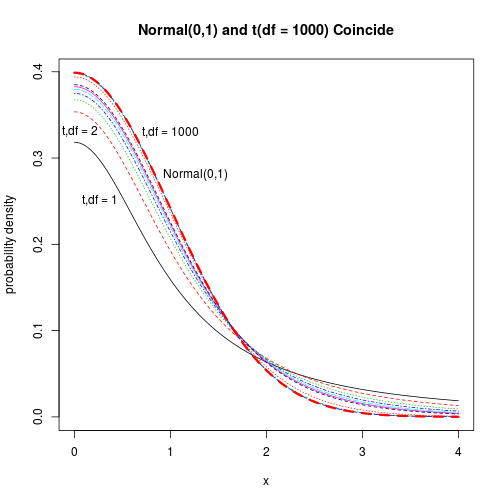``````if (SAVEME) dev.off()

## Now run the EXACT SAME THING and drop results into
## encapsulated postscript and png formats
if (SAVEME) {
postscript(file = "normalAndTcompared-1.eps", paper = "special",
onefile = FALSE, family = "Times", height = 7,
width = 7, horizontal = FALSE)
matplot(x,y,  type = "l", ylab = "probability density")
lines(x, dnorm(x), col = 2, lty = 2, lwd = 3)
text(0, 0.25, "t,df = 1", pos = 4)
text(1, 0.33, "t,df = 1000")
text(.85, 0.28, "Normal(0,1)", pos = 4)
text(-0.2, 0.33, "t,df = 2", pos = 4)
title("Normal(0,1) and t(df = 1000) Coincide")
dev.off()

png(file = "normalAndTcompared-1.png", width = 800, height = 800)
matplot(x,y,  type = "l", ylab = "probability density")
lines(x, dnorm(x), col = 2, lty = 2, lwd = 3)
text(0, 0.25, "t,df = 1", pos = 4)
text(1, 0.33, "t,df = 1000")
text(.85, 0.28, "Normal(0,1)", pos = 4)
text(-0.2, 0.33, "t,df = 2", pos = 4)
title("Normal(0,1) and t(df = 1000) Coincide")
dev.off()
}

### Now lets replicate a bunch of exponential means, compare against Normal
### and t.

### Note my code has a bunch of copied "stanzas" with just little changes.

### As I work my way down, I find ways to improve and beautify.
### If I kept working, I would revise to write a nice tight function
### And I would NOT have code copied over and over with little changes.
### But this is not bad for a first try.

if (SAVEME) pdf(file = "normalAndTcompared-2.pdf")
curve(dexp(x, rate = 0.410), from = 0, to = 40)
````````````par(mfrow = c(2,1))

myMeans <- replicate(100, mean(rexp(100, rate = 4.1)))
hist(myMeans, breaks = 50, prob = TRUE)
lines(density(myMeans))

newx <- seq(min(myMeans),max(myMeans),length.out = 100)

lines(newx, dnorm(newx, mean = mean(myMeans), sd = sd(myMeans)),lty = 3,
col = 3, lwd = 3)

myMeans <- replicate(100, mean(rexp(5000, rate = 0.41)))
hist(myMeans, breaks = 50, prob = TRUE)
lines(density(myMeans))

newx <- seq(min(myMeans),max(myMeans),length.out = 100)

lines(newx, dnorm(newx, mean = mean(myMeans), sd = sd(myMeans)),lty = 3,
col = 3, lwd = 3)
``````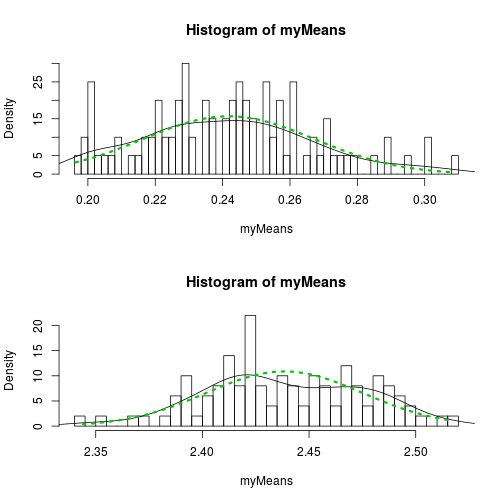``````curve(dexp(x, rate = 0.10), from = 0, to = 40)

myMeans <- replicate(100, mean(rexp(100, rate = 0.1)))
hist(myMeans, breaks = 50, prob = TRUE)
lines(density(myMeans))

newx <- seq(min(myMeans), max(myMeans), length.out = 100)
lines(newx, dnorm(newx, mean = mean(myMeans), sd = sd(myMeans)), lty = 3, col = 3, lwd = 3)
``````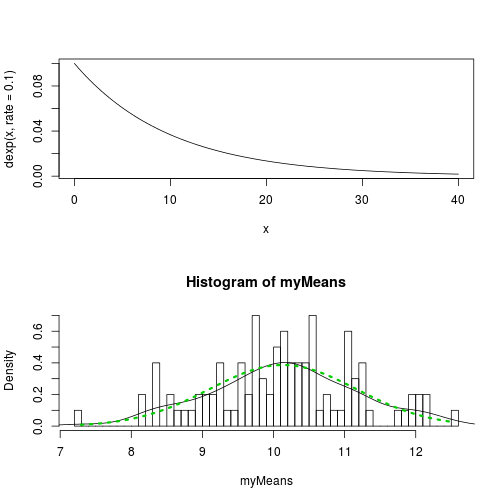``````myMeans <- replicate(100, mean(rexp(20000, rate = 0.1)))
hist(myMeans, breaks = 50, prob = TRUE)
lines(density(myMeans))

newx <- seq(min(myMeans),max(myMeans),length.out = 100)
lines(newx, dnorm(newx, mean = mean(myMeans), sd = sd(myMeans)),lty = 3, col = 3, lwd = 3)

### How to compare that against a t distribution?

### Found a nice routine in the car package.
library(car)
### Compare myMeans against t distribution with df = 2000
qqPlot(myMeans, distribution = "t",df = 2000)
``````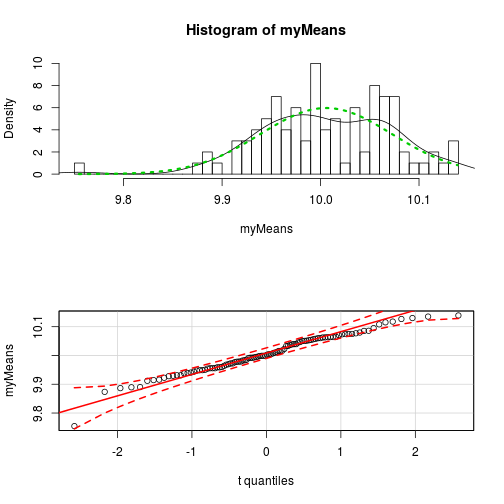``````### Compare myMeans against a Normal
qqnorm(myMeans)
qqline(myMeans)

curve(dexp(x, rate = 0.05), from = 0, to = 40)
````````````#### Try to make some nicer looking output, eh?  Start by comparing against
#### a Normal distribution that has its mean = observed mean of x and
#### std.dev. = observed std. dev.

#### I don't know why I made that comparison, I just did.

par(mfrow = c(3,1))

sampleSize <- 100
rate = 0.05
myMeans <- replicate(100, mean(rexp(sampleSize, rate = 0.05)))
hist(myMeans, breaks = 50, prob = TRUE,
main = paste("Histogram, Means from Exponential, rate = ",
rate, "N = ",sampleSize))
lines(density(myMeans))

newx <- seq(min(myMeans),max(myMeans),length.out = 100)

lines(newx, dnorm(newx, mean = mean(myMeans), sd = sd(myMeans)),
lty = 3, col = 3, lwd = 3)

lines(newx, dt(newx, df = 2),lty = 2, col = 2, lwd = 2)

sampleSize <- 1000
rate = 0.05
myMeans <- replicate(100, mean(rexp(sampleSize, rate = 0.05)))
hist(myMeans, breaks = 50, prob = TRUE,
main = paste("Histogram, Means from Exponential, rate = ",
rate, "N = ",sampleSize))
lines(density(myMeans))

newx <- seq(min(myMeans),max(myMeans),length.out = 100)
lines(newx, dnorm(newx, mean = mean(myMeans), sd = sd(myMeans)),lty = 3, col = 3, lwd = 3)

sampleSize <- 10000
rate = 0.05
myMeans <- replicate(100, mean(rexp(sampleSize, rate = 0.05)))
hist(myMeans, breaks = 50, prob = TRUE,
main = paste("Histogram, Means from Exponential, rate = ",
rate, "N = ",sampleSize))
lines(density(myMeans))

newx <- seq(min(myMeans),max(myMeans),length.out = 100)
lines(newx, dnorm(newx, mean = mean(myMeans), sd = sd(myMeans)),lty = 3, col = 3, lwd = 3)
``````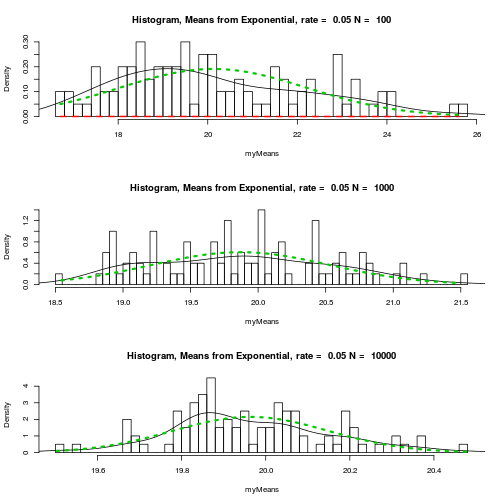``````qqPlot(myMeans, distribution = "t",df = 20)

sampleSize <- 100
rate = 0.05
myMeans <- replicate(100, mean(rexp(sampleSize, rate = 0.05)))
### Convert to t-like value
myMeans <-(myMeans - mean(myMeans))/sd(myMeans)
hist(myMeans, breaks = 50, prob = TRUE,
main = paste("Histogram, Standardized Means from Exponential, rate = ",
rate, "N = ", sampleSize))
lines(density(myMeans))

newx <- seq(min(myMeans),max(myMeans),length.out = 100)

### If you set the t's df = sample size, it is just about "right on"
lines(newx, dt(newx, df = sampleSize),lty = 2, col = 2, lwd = 2)

sampleSize <- 1000
rate = 0.05
myMeans <- replicate(100, mean(rexp(sampleSize, rate = 0.05)))
### Convert to t-like value
myMeans <-(myMeans - mean(myMeans))/sd(myMeans)

hist(myMeans, breaks = 50, prob = TRUE, main =
paste("Histogram, Standardized Means from Exponential, rate = ",
rate, "N = ",sampleSize))
lines(density(myMeans))

newx <- seq(min(myMeans),max(myMeans),length.out = 100)

### If you set the t's df = sample size, it is just about "right on"
lines(newx, dt(newx, df = sampleSize),lty = 2, col = 2, lwd = 2)
``````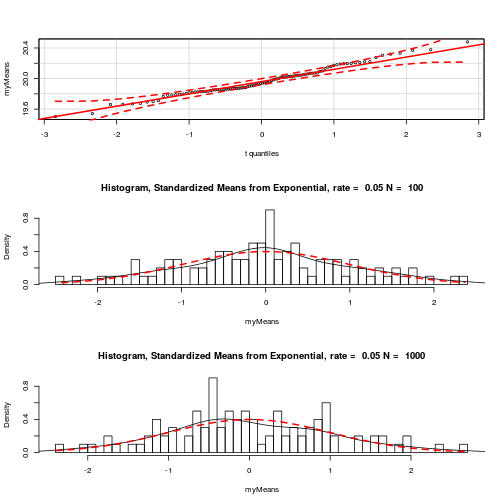``````sampleSize <- 5000
rate = 0.05
myMeans <- replicate(100, mean(rexp(sampleSize, rate = 0.05)))
### Convert to t-like value
myMeans <-(myMeans - mean(myMeans))/sd(myMeans)
hist(myMeans, breaks = 50, prob = TRUE, main =
paste("Histogram, Standardized Means from Exponential, rate = ",
rate, "N = ",sampleSize))
lines(density(myMeans))

newx <- seq(min(myMeans),max(myMeans),length.out = 100)

### If you set the t's df = sample size, it is just about "right on"
lines(newx, dt(newx, df = sampleSize),lty = 2, col = 2, lwd = 2)

if (SAVEME) dev.off()
``````